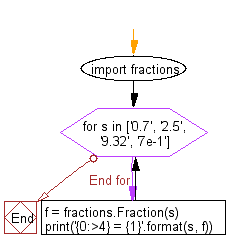﻿ Python Math: Display the fraction instances of the string representation of a number - w3resource# Python Math: Display the fraction instances of the string representation of a number

## Python Math: Exercise-43 with Solution

Write a Python program to display the fraction instances of the string representation of a number.

Sample Solution:-

Python Code:

``````import fractions

for s in ['0.7', '2.5', '9.32', '7e-1']:
f = fractions.Fraction(s)
print('{0:>4} = {1}'.format(s, f))
```
```

Sample Output:

``` 0.7 = 7/10

2.5 = 5/2

9.32 = 233/25

7e-1 = 7/10
```

Flowchart:Python Code Editor:

Have another way to solve this solution? Contribute your code (and comments) through Disqus.

What is the difficulty level of this exercise?

Test your Programming skills with w3resource's quiz.

﻿

```>>> students = [{'name': 'John', 'score': 98}, {'name': 'Mike', 'score': 94}, {'name': 'Jennifer', 'score': 99}]